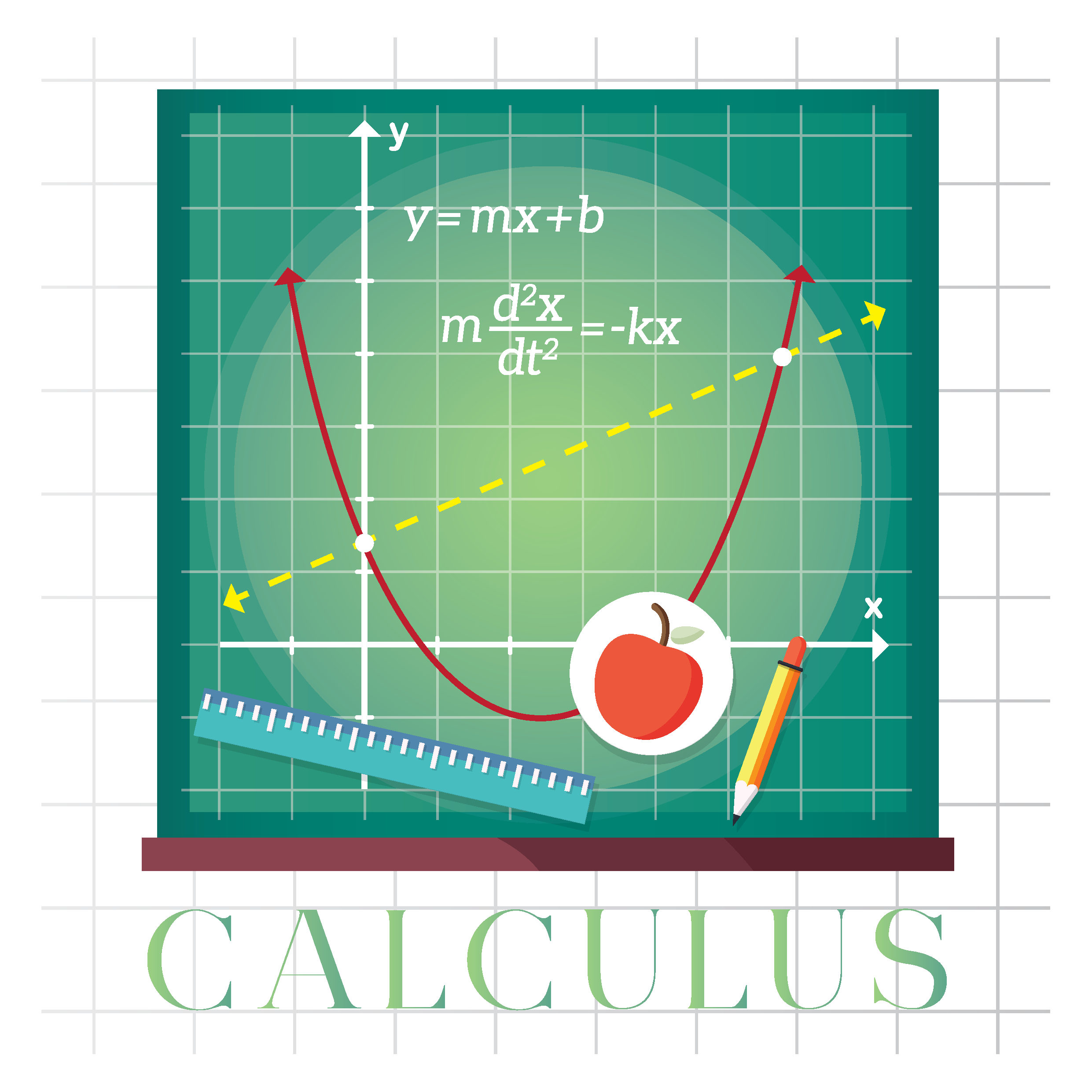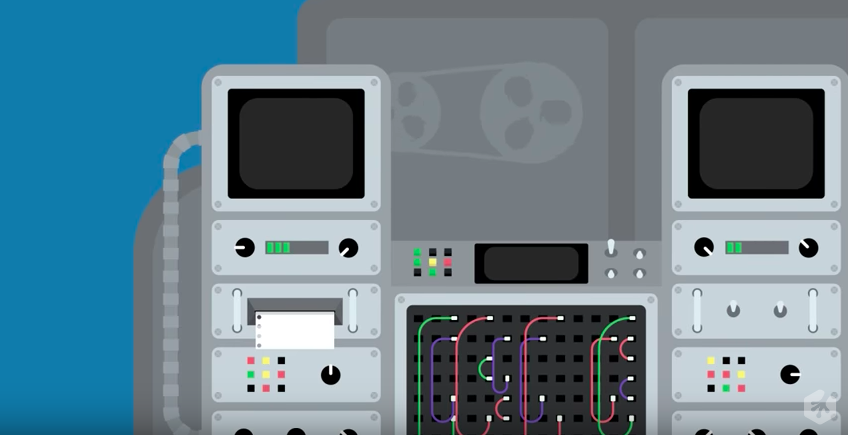We use cookies and other tracking technologies to improve your browsing experience on our site, analyze site traffic, and understand where our audience is coming from. To find out more, please read our privacy policy.

By choosing 'I Accept', you consent to our use of cookies and other tracking technologies.

We use cookies and other tracking technologies to improve your browsing experience on our site, analyze site traffic, and understand where our audience is coming from. To find out more, please read our privacy policy.

By choosing 'I Accept', you consent to our use of cookies and other tracking technologies. Less

We use cookies and other tracking technologies... More

# Login or registerto boost this post!

Show some love to the author of this blog by giving their post some rocket fuel 🚀.

# Login or register to start working on this issue!

Engineers who find a new job through Functional Works average a 15% increase in salary 🚀# Lambda Calculus In Clojure - Part 1

Lambda Calculus is the smallest programming language. As we saw on my previous post, the only building blocks available are functions, variables and expressions. There are no built-in primitive values or operations. How can we then solve real-world problems using Lambda Calculus?

In this post, we are going to create a set of building blocks, using lambda expressions, to calculate boolean expressions. For this, we are going to use Clojure, which is a dynamic functional language based on Lambda Calculus.

## Church Encoding

A mathematician called Alonzo Church was able to encode data and operators in Lambda Calculus. Church encoding is the name of this way of building primitive terms and operations, converting them into higher-order functions that can be combined to create more complex expressions.

There are a few different primitives that can be constructed in Church Encoding:

• Booleans
• Integers
• Pairs
• Lists
• Tagged unions

Each of these primitives have their own operators, that can be built in a similar way to the values of the primitives. This gives us the basic building blocks for writing programs in Lambda Calculus. For the rest of this post, we are going to focus on Church booleans.

### Church booleans

In boolean logic, there are only two possible values, `true` and `false`. As we saw previously, Lambda Calculus doesn’t provide any primitive values, but we can define them in Church Encoding. Given a lambda function with two parameters, we define `true` and `false` as following:

``````true = λa.λb.a
false = λa.λb.b
``````

Looking at this definition, we can see that `true` is a function of two parameters that returns the first one. Similarly, `false` returns the second parameter.

Apart from primitive values, we need some operators in order to complete a boolean algebra and write programs. We can define the boolean operators based on the definitions for `true` and `false` above:

``````and = λp.λq.p q p
or = λp.λq.p p q
``````

`and` returns q if p is `true`; otherwise, returns p, which equals to `false`. We can use the definition of `true` and `false` above to see the substitution in detail:

When p is true:

``````p = true = λa.λb.a
and = λp.λq.p q p = λp.λq.((λa.λb.a)(q p)) = λp.λq.q = q
``````

When p is false:

``````p = false = λa.λb.b
and = λp.λq.p q p = λp.λq.((λa.λb.b)(q p)) = λp.λq.p = p = false
``````

`or` works in a similar way.

``````not = λp.p false true
``````

Applying the same reasoning, `not` just inverts the value of p, returning `false` if p is `true`, and returning `true` otherwise.

The last operator is Exclusive OR:

``````xor = λa.λb.a (not b) b
``````

which follows the same rules as the previous operators.

It’s also possible to define an `if` predicate, similar to the ones we use in most programming languages:

``````if = λp.λa.λb.p a b
``````

The predicate p is applied to the arguments a and b, returning a if the predicate evaluates to `true`, and b if the predicate evaluates to `false`.

## Creating the building blocks

After defining the boolean algebra in Church Encoding, we are ready to start building it in Clojure.

### Lambdas

Clojure has built-in anonymous functions, which can be used to simulate functions in Lambda Calculus. For example, the identity function would look like this:

``````(fn [x] x)
``````
• `fn` is the anonymous function definition.
• `[x]` is the parameter of the function.
• `x` is the body of the function.

Does this look familiar? We could rewrite this expression in Lambda Calculus:

``````(λx.x)
``````

Both expressions look quite similar. This is because Clojure is based on Lambda Calculus. But, what if we could add some syntactic sugar to the recipe and make Clojure’s anonymous functions even closer to their Lambda Calculus counterparts?

### Defining a λ-macro

Macros in Clojure are extremely powerful. They are used to extend the language. Let’s redefine the anonymous function as a λ function:

``````(defmacro λ
[args & body]
`(fn [~args] ~@body))
``````

This macro will take the first element after λ and make it the single argument of an anonymous function, which will take the rest of the form as the body. This allows us to rewrite the original identity function `(fn [x] x)` as `(λ x x)`, which is very close to the Lambda Calculus syntax `(λx.x)`.

Now, we can start building the boolean algebra using our new shiny syntax.

### Booleans

Let’s start with `true` and `false`. As they are reserved words in Clojure, we are going to rename them to `T` and `F`:

``````; true = λa.λb.a
(def T
(λ a (λ b a)))

; false = λa.λb.b
(def F
(λ a (λ b b)))
``````

Notice that we are using `def` instead of `defn`, to define the term, which is a higher-order function (returning a function, in this case). The difference between them is that `defn` will be evaluated every time it’s called, whereas `def` will only be evaluated once. This is not strictly necessary for our purposes, but doing so, we can reinforce the idea that lambda expressions always return the same result when evaluated.

The boolean operators implementation follows a similar idea to `true` and `false`:

``````; and = λp.λq.p q p
(def And
(λ p (λ q ((p q) p))))

; or = λp.λq.p p q
(def Or
(λ p (λ q ((p p) q))))

; not = λp.λa.λb.p false true
(def Not
(λ p ((p F) T)))

; xor = λa.λb.a (not b) b
(def Xor
(λ a (λ b ((a (Not b)) b))))

; if = λp.λa.λb.p a b
(def If
(λ p (λ a (λ b ((p a) b)))))
``````

## A boolean calculator

Now that we have implemented our boolean algebra, we can start evaluating boolean expressions. In order to help us check the results, we can add a function to convert a λ-boolean into a Clojure boolean:

``````(def toBoolean
(λ f ((f true) false)))
``````

Let’s try to evaluate a few expressions:

``````; true AND true
=> (toBoolean ((And T) T))
true
``````

Similarly,

``````; true AND false
=> (toBoolean ((And T) F))
false
``````

To evaluate more complex expressions, we just need to nest boolean expressions:

``````(deftest λ-booleans
(testing "Boolean expressions"
;T AND T AND F OR T
(is (= (toBoolean ((And T) ((And T) ((Or F) T))))
true))

;T AND F AND F OR T AND T
(is (= (toBoolean ((And T) ((And F) ((Or F) ((And T) T)))))
false))

;T OR (F AND F OR T AND T)
(is (= (toBoolean ((Or T) ((And F) ((Or F) ((And T) T)))))
true))

;F OR (F AND F OR F AND T)
(is (= (toBoolean ((Or F) ((And F) ((Or F) ((And F) T)))))
false))

;F OR F AND F OR T AND T
(is (= (toBoolean ((And ((Or F) F)) ((And T) ((Or F) T))))
false))

;T OR F AND F OR T AND T
(is (= (toBoolean ((And ((Or T) F)) ((And T) ((Or F) T))))
true))))
``````

You can find the source code here.

## Conclusion

The Church-Turing Thesis states that any computation that is Turing computable can be represented using Church encoding. We’ve seen how to do this for boolean expressions. In part 2, we will implement a numerals algebra.

## References

Church Encoding

http://blog.errstr.com/2012/01/14/church-encoding/

Church-Turing Thesis

Programming With Nothing by Tom StuartOriginally published on srodrigo.me